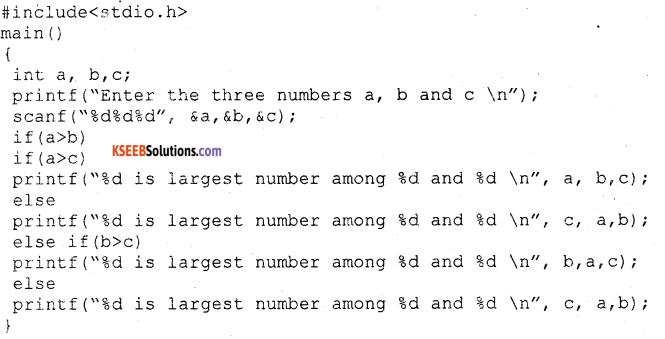# 2nd PUC Electronics Previous Year Question Paper March 2019

Students can Download 2nd PUC Electronics Previous Year Question Paper March 2019, Karnataka 2nd PUC Electronics Question Bank with Answers helps you to revise the complete Karnataka State Board Syllabus and score more marks in your examinations.

## Karnataka 2nd PUC Electronics Previous Year Question Paper March 2019

### 2nd PUC Electronics Previous Year Question Paper March 2019

Time : 3 hrs. 15 min.
Max Marks: 70

Instructions:

• The question has five Parts A, B, C and D.
• Part – A has no choice
• Part – D has two parts. Part -1 is from problems, Part – II is of essay type questions.
• Circuit diagram/timing diagram/truth tables are drawn wherever necessary.
• Problems without necessary formula/formulae carry no marks.

Part – A

Answer all questions: 10 x 1 = 10

Question 1.
Name the charge carriers in N-channel JFET.
Electrons.

Question 2.
Write the value of bandwidth of an ideal OPAMP.
Infinity.

Question 3.
Draw the waveform of damped oscillations.Question 4.
What is the function of transmitter in a radio communication system?
Transmitter processes the information and transmits it.Question 5.
Draw the waveform of AM wave when modulation index ma=1Question 6.
Fidelity is the ability of the receiver to reproduce the input faithfully.

Question 7.
Mention one application of TWAC.
TRIAC can be used in fan motor control.

Question 8.
Write the decimal equivalent of BCD code (00110101)2.
(00110101)2 = 3510.

Question 9.
Name anyone arithmetic operator in C-language.Question 10.
Expand RADAR. or = r v                                                                       ‘

Part – B

Question 11.
Mention the steps to obtain DC-equivalent circuit of a CE amplifier.

• Reduce all ac sources to ground.
• Open all the capacitors.

Question 12.
Calculate the gain of a negative feedback amplifier with an internal gain, A = 100 and feedback factor β= 1/10.
A= 100, β = 1/10.Question 13.
Write any two applications of crystal oscillator.

• Digital watches.
• Clock, Calculators.

Question 14.
Realise XOR gate using basic logic gates.Question 15.
Write the difference between combinational and sequential logic circuits.

 Combinational Logic Circuit Sequential Logic Circuit (i)  It does not have memory (ii)  Its output depends only on present input. (i) It has memory. (ii) Its output depends on present and past inputQuestion 16.
Write any two instruction set of 8051 microcontroller.
These instructions move the content of one register to another one. Data can also be transferred to stack with the help of PUSH and POP instructions.Question 17.
Mention any two features of C-language.
C is a powerful, flexible language that has gained worldwide acceptance in recent years. C is concise, yet powerful in its scope. It is a high-level language that also incorporates features of low-level languages. It is becoming the standard for program development on small machines and microcomputers.

It is possible to apply C to nearly all application areas. Modularity {process of dividing problems into sub-problems) makes C ideal for projects involving several programmers. C permits nearly every programming technique. C is widely available for most brands and type of computers. It is portable. With all the features C is not a difficult language, it is one of the easiest languages to learn and implement.

C programming can be used to write any complex program because of its rich set of built-in functions and operations. C compiler combines the capabilities of an assembly language with the feature of high-level language and therefore it is very much suited for uniting both system software and business packages. Because of its variety of data types, powerful operator programs in C are efficient and fast. C has the ability to extend, it means user-defined functions can also be added to C library.Question 18.
Briefly explain the internet.

1. Using internet, access to required information is easy, economical, reliable and fast.
2. With E-mail, messages reach destination with in minutes.
3. E-mail can be sent and received from any place.
4. Using E-mail, message can be sent to several persons simultaneously.

Part – C

Answer any five questions.   5 x 3 = 15

Question 19.
Compare the FET and BJT.

 FET BJT. 1. It is unipolar device. 1. It is a bipolar device. 2. It is a voltage controlled device. 2. It is a current controlled devices. 3. Its input resistance is very high. 3. Input resistance is very low. 4. Current conduction is only by majority charge carriers either hole or electron. 4. Current conduction is both by electrons and holes. 5. It has high switching speed. 5. It has low switching speed. 6. Less noisy 6. More noisy. 7. Fabrication is easier in IC. 7. Difficult to fabricate in IC.

Question 20.
What is transistor biasing? Briefly explain the need for biasing a transistor
The application of suitable DC voltage across the transistor terminals is called biasing of the transistor.

The reasons for biasing of a transistor are:

1. To make the operating point to be at the centre of the load line.
2. To make the collector current independent of temperature variations.
3. To maintain the operating point constant even though transistor is replaced by another of same type.Question 21.
With a block diagram derive an expression for output impedance of aN amplifer with negative feedback.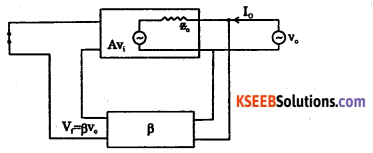An amplifier should have a low output impedance so that it can deliver power to load without much loss. With negative feedback, the output impedance of the amplifier decreases which is desirable.

Let Zo be output impedance of basic amplifier and Zof be the output impedance with negative feedback. A hypothetical source of voltage Vo is applied at the output. By applying KVL to the output loop,

Vo = IoZo +AVi.
With negative feedback, input to basic amplifier with negative feedback is
Vi = Vs – Vf
V= o – Vf
V– Vf
{As input is short circuited, V = o}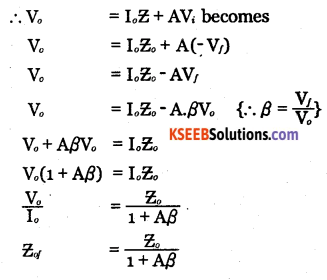As 1+Aβ>1, output impedance of amplifier decreases by a factor(1+Aβ)

Question 22.
Explain the importance of ionosphere in the radio communication.
Ultraviolet radiation from the sun cause the upper atmosphere to ionize i.e. to become electrically charged. Hence a thick layer of ions formed at heights of 50 km to 400km. The ionosphere supports MF and HF wave propagation.
Its three layers are:

D layer: It is at a height of 70 km and has an average thickness of 10 km. It disappears in the night time. It reflects VLF and LF waves and absorbs MF and HF waves.

E layer: It is at a height of 100 km with a thickness of 25 km. This layer disappears at night. It helps in MF and HF wave propagation.

F layer: It is at a height of 150 km and extends upto 400 km. This layer exists during both day and night time. During day time, it splits into two layers F, and F2, and combines to form a single F layer during night time.

 F2 region. 250-400 km. F1 region. 160-250 km. E region. 95-130 km D region. 50-95 km. Troposphere Earth.Question 23.
Derive an expression for the total power of AM wave.
The total power in AM wave is equal to the sum of powers of the carrier, the upper sideband and the lower sideband.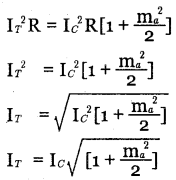Question 24.
In a SCR full wave rectifier the firing angle is 90°, rms voltage of an input to the rectifier is 230 V and the load resistance is 25Ω. Calculate Vdc and Idc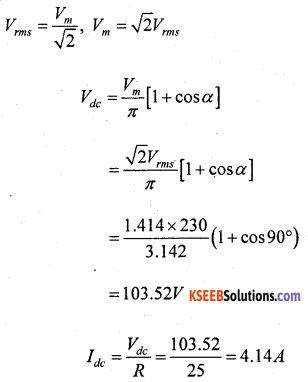Question 25.
With a neat circuit diagram explain the working of power diode under forward bias condition.Question 26.
With a neat block diagram explain the satellite Transponder.Part – D

Answer any Three question  3 x 5 = 15

Question 27.
Calculate voltage gain (Ay) and input impedence (Zin) of a given CE amplifer with values
$$r_{ e }=\frac { 26{ mV } }{ { I }_{ { E } } } ,{ V }_{ { BE } }=0.7V$$ β = 100.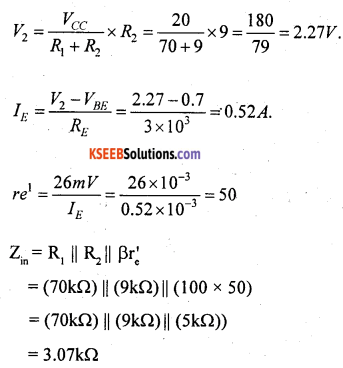Question 28.
Calculate the output voltage (V0) for a given circuit below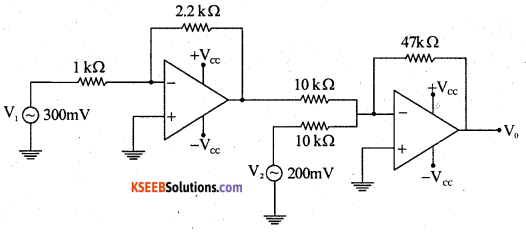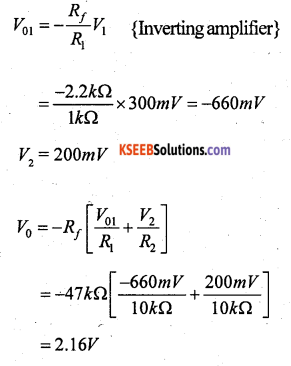Question 29.
A transistor Colpitts oscillator has L = 4 mH, C1 = 10 nF and C2 = 10 nF. Calculate the frequency of oscillations.Question 30.
The current of a AM transmitter is 8A when the carrier is sent, it increases to 8.65 A when the carrier is modulated. Find the percentage of modulation. Also calculate the antenna current when the depth of modulation is 0.75.Question 31.
Simplify the Boolean expression using K-map. Y(ABCD) = Σm(0, 2, 4, 5, 8, 10, 12, 14) + Σd(6, 13)  Write the logic circuit for the simplified expression.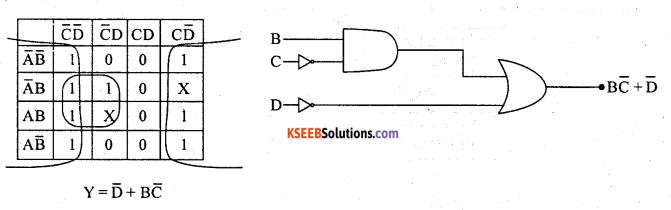Part – E

Question 32.
Compare CE, CB and CC amplifiers.

 Parameter Types of Amplifiers. CE CB CC Current gain High (P) Less than 1 Highest (1+ p) Voltage gain High Moderate Low Power gain Highest Moderate Moderate Phase shift 180° 0° 0- Input impedance Moderate Low High Output impedance Moderate High Low Bandwidth Narrow Wide Wide Applications Used as AF amplifies Used as RF amplifies impedance matchingQuestion 33.
(a) What are active filters? (1 + 4)
An active filter is a type of analog circuit implementing an electronic filter using active components, typically an amplifier. Amplifiers included in a filter design can be used to improve the cost, performance and predictability of a filter.

(b) With a neat circuit diagram explain the working of first order low pass active filter and draw its frequency response curve.
Filter are circuits used to select a band of frequencies. The two types of filters are

• low pass filter and
• high pass filter.Cut off frequency $$\mathrm{f}_{C}=\frac{1}{2 \Pi \mathrm{R}_{1} \mathrm{C}_{1}}$$
Frequency response of low pass filter.

Question 34.
Draw a block diagram and explain the different stages of FM transmitter.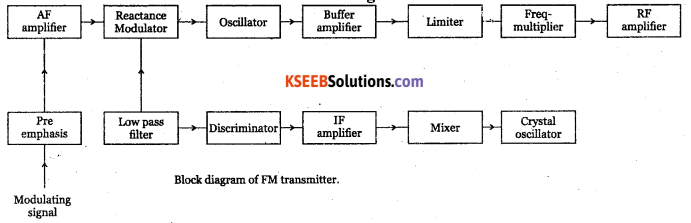The modulating signal is applied to the pre-emphasis circuit, which improves signal to noise ratio, AF amplifier amplifies output of pre-emphasis. The processed signal is fed to reactance modulator. The reactance modulator uses a transistor or FET connected across tank circuit of carrier oscillator.

The oscillator frequency depends on the tank reactance which in turn depends on the instantaneous amplitude of modulating signal. Thus, output of reactance modulator will be a frequency modulated wave.

The oscillator is followed by a buffer amplifier which isolates oscillator from subsequent stages. The limiter maintains the amplitude constant. Class C power amplifier amplifies modulated wave to required power levels. The FM signal is then fed to the transmitting antenna.

Question 35.
(a)What is flip-flop?
A flip flop is an electronic circuit with two stable states that can be used to store binary data. Flip-flops and latches are fundamental building blocks of digital electronics systems used in computers, communications, and many other types of systems. Flip-flops and latches are used as data storage elements

(b) With a logic diagram and truth table, explain the working of SR flip-flop, (1+4)Question 36.
Write a flow chart and explain the steps used in creating assembly language program in 8051 microcontroller